# 吴恩达《Machine Learning》精炼笔记 8：聚类 KMeans 及其 Python实现

• 算法思想
• 图解K-Means
• sklearn实现
• Python实现

## 无监督学习unsupervised learning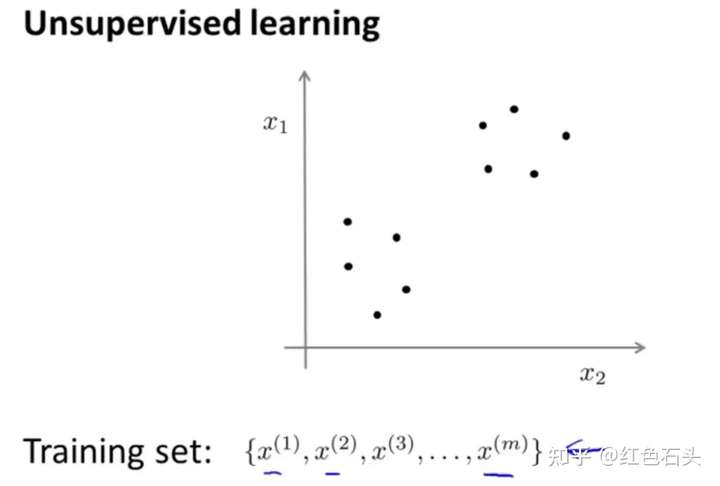• 市场分割
• 社交网络分析
• 组织计算机集群
• 了解星系的形成1.Jaccard 系数
2.FM 系数
3.Rand 系数

1.DB指数
2.Dunn指数

DBI的值越小越好，Dunn的值越大越好。

xi,xj 的Lp的距离定义为：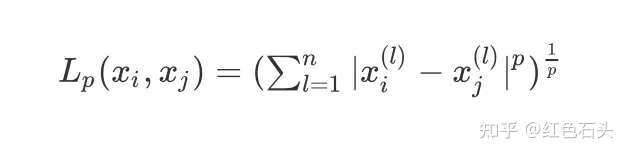• 当p=2时，即为欧式距离，比较常用，即：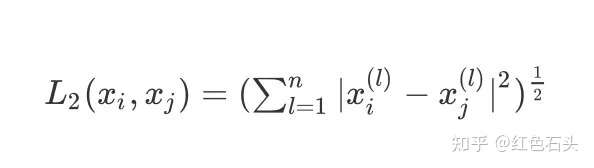• 当p=1时，即曼哈顿距离，即：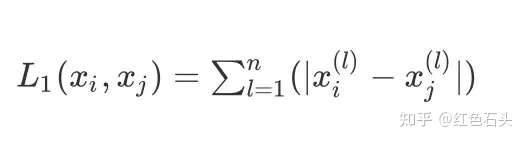• 当p趋于无穷，为切比雪夫距离，它是各个坐标距离的最大值：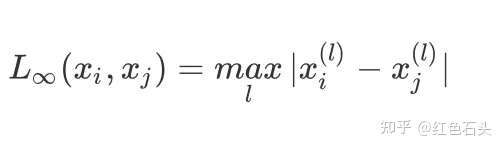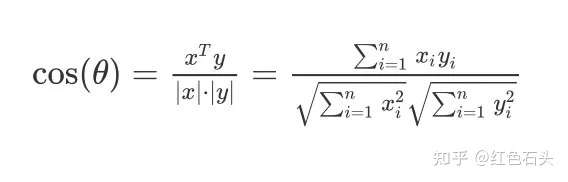Pearson皮尔逊相关系数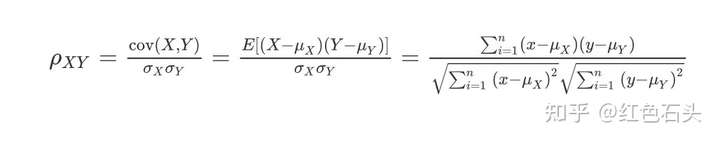## K-均值算法

K-均值，也叫做k-means算法，最常见的聚类算法，算法接受一个未标记的数据集，然后将数据聚类成不同的组。假设将数据分成n个组，方法为：

• 随机选择K个点，称之为“聚类中心”
• 对于数据集中的每个数据，按照距离K个中心点的距离，将其和距离最近的中心点关联起来，与同个中心点关联的所有点聚成一类。
• 计算上面步骤中形成的类的平均值，将该组所关联的中心点移动到平均值的位置
• 重复上面两个步骤，直到中心点不再变化。

1.给定需要划分的数据，随机确定两个聚类中心点
2.计算其他数据和这两个中心点的距离，划入距离小的类中，假设两个类是C1,C2
3.确定上述步骤中两个类是C1,C2的均值，这个均值就是新的聚类中心
4.重复：计算数据和这两个中心点的距离，划入距离小的类中，形成新的类；再确定新的聚类中心
5.直至中心点不再变化，结束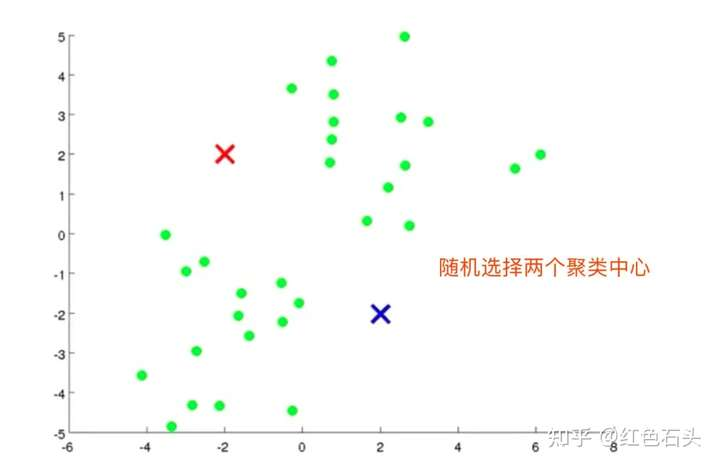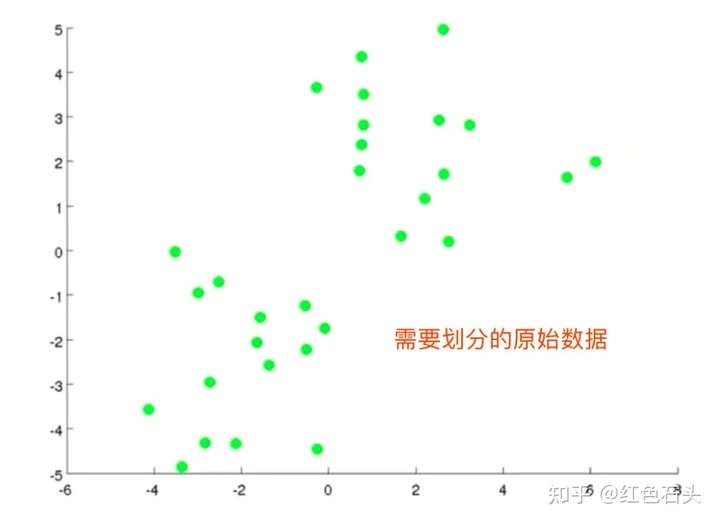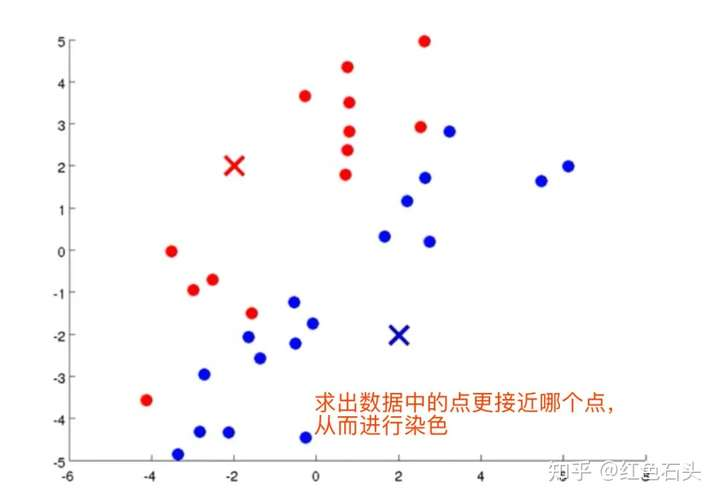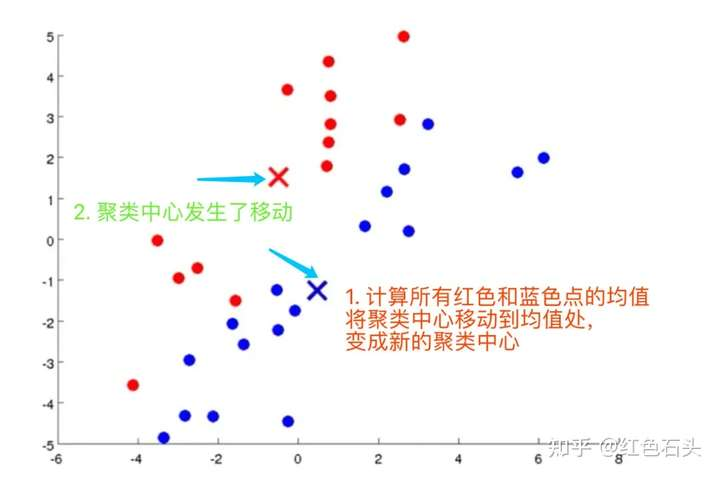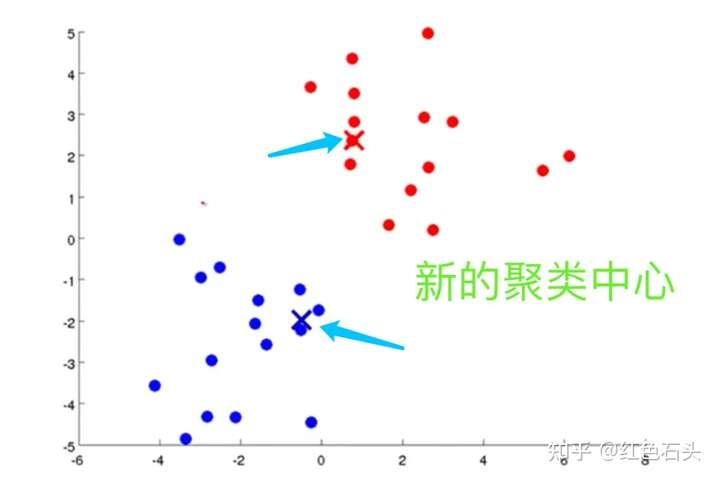K-means算法过程

repeat {
for i= to m
#  计算每个样例属于的类
c(i) := index (from 1 to K)  of cluster centroid closest to x(i)

for k = 1 to K
# 聚类中心的移动，重新计算该类的质心
u(k) := average (mean) of points assigned to cluster K
}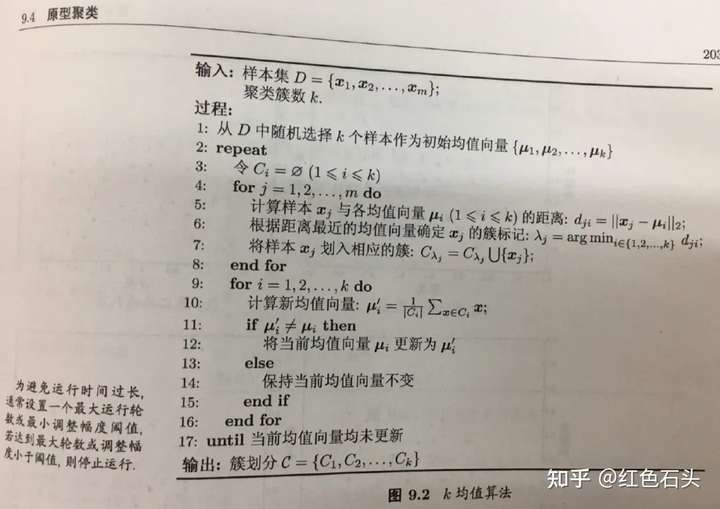K-均值最小化问题，是要最小化所有的数据点与其所关联的聚类中心点之间的距离之和，因此 K-均值的代价函数（畸变函数Distortion function） ：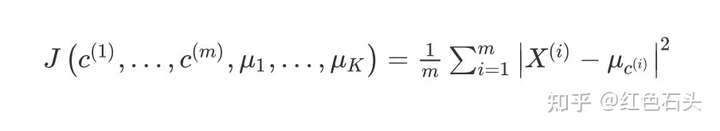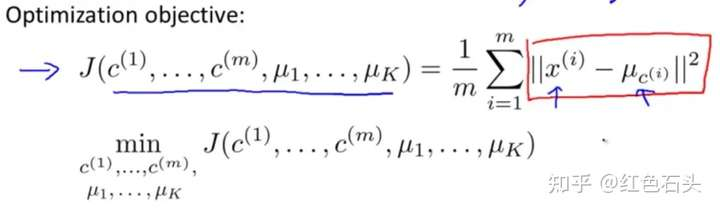• 选择K<m，即聚类中心的个数小于训练样本的实例数量
• 随机训练K个训练实例，然后令K个聚类中心分别和这K个训练实例相等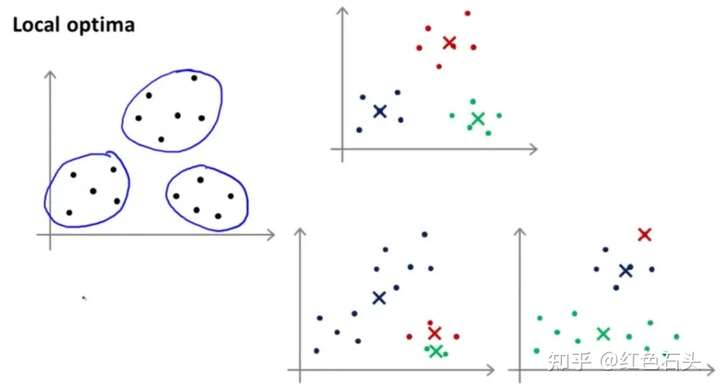## Scikit learn 实现K-means

make_blobs数据集

make_blobs聚类数据生成器make_blobs方法常被用来生成聚类算法的测试数据。它会根据用户指定的特征数量、中心点数量、范围等来生成几类数据。

sklearn.datasets.make_blobs(n_samples=100, n_features=2,centers=3, cluster_std=1.0, center_box=(-10.0, 10.0), shuffle=True, random_state=None)[source]

• n_samples是待生成的样本的总数
• n_features是每个样本的特征数
• centers表示类别数
• cluster_std表示每个类别的方差
import numpy as np
import matplotlib.pyplot as plt
# 导入 KMeans 模块和数据集
from sklearn.cluster import KMeans
from sklearn.datasets import make_blobs

# 定义画布
plt.figure(figsize=(12,12))

# 定义样本量和随机种子
n_samples = 1500
random_state = 170

# X是测试数据集，y是目标分类标签0，1，2
X, y = make_blobs(n_samples=n_samples, random_state=random_state)

X
array([[-5.19811282e+00,  6.41869316e-01],
[-5.75229538e+00,  4.18627111e-01],
[-1.08448984e+01, -7.55352273e+00],
...,
[ 1.36105255e+00, -9.07491863e-01],
[-3.54141108e-01,  7.12241630e-01],
[ 1.88577252e+00,  1.41185693e-03]])

y
array([1, 1, 0, ..., 2, 2, 2])

# 预测值的簇类
y_pred = KMeans(n_clusters=2, random_state=random_state).fit_predict(X)
y_pred
array([0, 0, 1, ..., 0, 0, 0], dtype=int32)

X[:,0]  # 所有行的第1列数据
array([ -5.19811282,  -5.75229538, -10.84489837, ...,   1.36105255,
-0.35414111,   1.88577252])

# 子图1的绘制
plt.subplot(221)
plt.scatter(X[:, 0], X[:, 1], c=y_pred)
plt.title("incorrrect Number of Blods")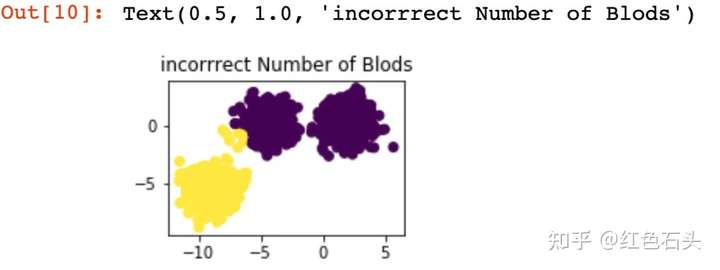transformation = [[0.60834549, -0.63667341],[-0.40887718, 0.85253229]]
X_aniso = np.dot(X, transformation)
y_pred = KMeans(n_clusters=3, random_state=random_state).fit_predict(X_aniso)
# 子图2的绘制
plt.subplot(222)
plt.scatter(X_aniso[:, 0], X_aniso[:, 1], c=y_pred)
plt.title("Anisotropicly Distributed Blobs")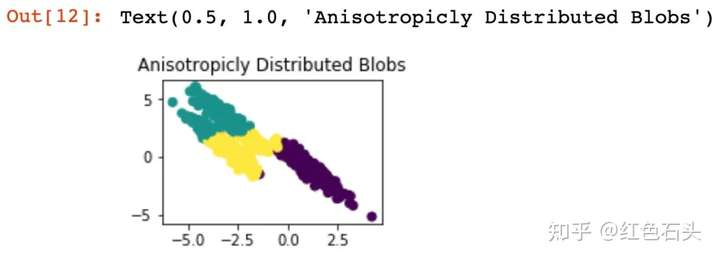X_varied, y_varied = make_blobs(n_samples=n_samples,
cluster_std=[1.0,2.5,0.5],random_state=random_state)
y_pred = KMeans(n_clusters=3, random_state=random_state).fit_predict(X_varied)
plt.subplot(223)
plt.scatter(X_varied[:, 0], X_varied[:, 1], c=y_pred)
plt.title("Unequal Variance")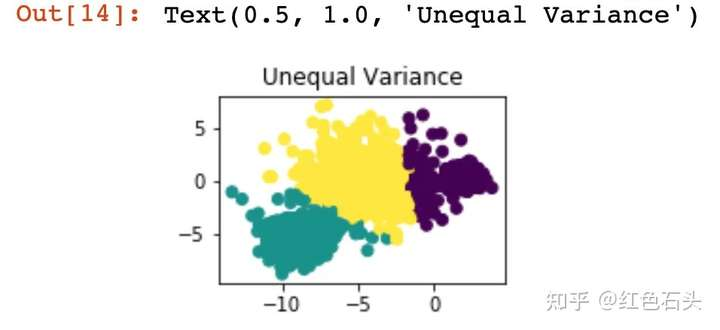X_filtered = np.vstack((X[y == 0][:500],
X[y == 1][:100],
X[y == 2][:10]))
y_pred = KMeans(n_clusters=3,random_state=random_state).fit_predict(X_filtered)
plt.subplot(224)
plt.scatter(X_filtered[:, 0],
X_filtered[:, 1],
c=y_pred)
plt.title("Unevenly Sized Blobs")
plt.show()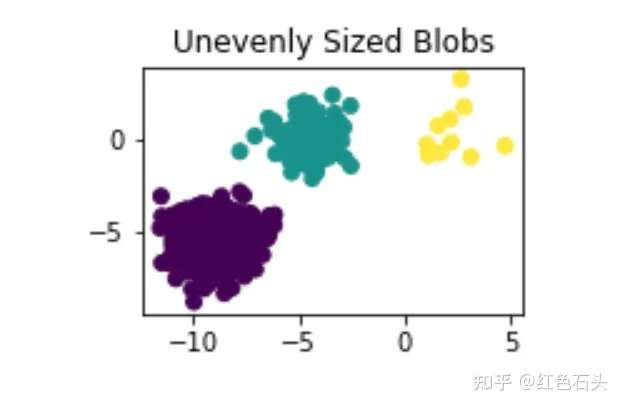## 基于 python实现K-means算法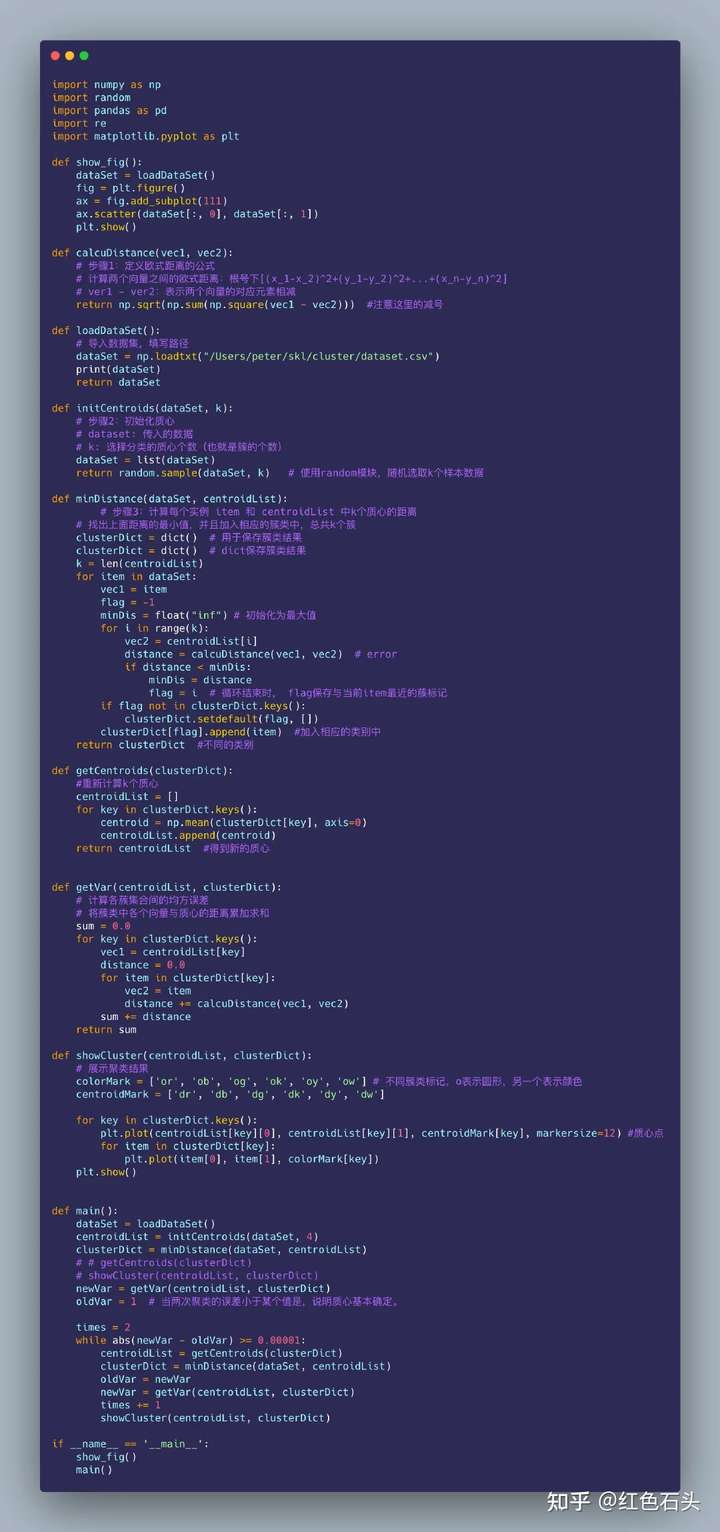### 觉得文章有用就打赏一下文章作者

#### 支付宝扫一扫打赏#### 微信扫一扫打赏